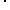# MINLPLib

### A Library of Mixed-Integer and Continuous Nonlinear Programming Instances

#### Instance: pooling_haverly2pq

`PQ formulation of pooling problem. Explicitly added RLT constraints were removed from the original formulation of Alfaki and Haugland.`
 Formatsⓘ ams gms lp mod nl osil pip Primal Bounds (infeas ≤ 1e-08)ⓘ -600.00000000 p1 ( gdx sol ) (infeas: 0) Other points (infeas > 1e-08)ⓘ Dual Boundsⓘ -600.00000060 (ANTIGONE)-600.00000180 (BARON)-600.00000000 (COUENNE)-600.00000000 (LINDO)-600.00003080 (SCIP) Referencesⓘ Haverly, C A, Studies of the Behavior of Recursion for the Pooling Problem, ACM SIGMAP Bull, 25, 1978, 19-28.Alfaki, Mohammed and Haugland, Dag, Strong formulations for the pooling problem, Journal of Global Optimization, 56:3, 2013, 897-916. Sourceⓘ Haverly2.gms from Standard Pooling Problem Instances Applicationⓘ Pooling problem Added to libraryⓘ 12 Sep 2017 Problem typeⓘ QCP #Variablesⓘ 10 #Binary Variablesⓘ 0 #Integer Variablesⓘ 0 #Nonlinear Variablesⓘ 4 #Nonlinear Binary Variablesⓘ 0 #Nonlinear Integer Variablesⓘ 0 Objective Senseⓘ min Objective typeⓘ linear Objective curvatureⓘ linear #Nonzeros in Objectiveⓘ 6 #Nonlinear Nonzeros in Objectiveⓘ 0 #Constraintsⓘ 13 #Linear Constraintsⓘ 9 #Quadratic Constraintsⓘ 4 #Polynomial Constraintsⓘ 0 #Signomial Constraintsⓘ 0 #General Nonlinear Constraintsⓘ 0 Operands in Gen. Nonlin. Functionsⓘ Constraints curvatureⓘ indefinite #Nonzeros in Jacobianⓘ 36 #Nonlinear Nonzeros in Jacobianⓘ 8 #Nonzeros in (Upper-Left) Hessian of Lagrangianⓘ 8 #Nonzeros in Diagonal of Hessian of Lagrangianⓘ 0 #Blocks in Hessian of Lagrangianⓘ 1 Minimal blocksize in Hessian of Lagrangianⓘ 4 Maximal blocksize in Hessian of Lagrangianⓘ 4 Average blocksize in Hessian of Lagrangianⓘ 4.0 #Semicontinuitiesⓘ 0 #Nonlinear Semicontinuitiesⓘ 0 #SOS type 1ⓘ 0 #SOS type 2ⓘ 0 Infeasibility of initial pointⓘ 1 Sparsity JacobianⓘSparsity Hessian of Lagrangianⓘ```\$offlisting
*
*  Equation counts
*      Total        E        G        L        N        X        C        B
*         14        6        0        8        0        0        0        0
*
*  Variable counts
*                   x        b        i      s1s      s2s       sc       si
*      Total     cont   binary  integer     sos1     sos2    scont     sint
*         11       11        0        0        0        0        0        0
*  FX      0
*
*  Nonzero counts
*      Total    const       NL      DLL
*         43       35        8        0
*
*  Solve m using NLP minimizing objvar;

Variables  objvar,x2,x3,x4,x5,x6,x7,x8,x9,x10,x11;

Positive Variables  x2,x3,x4,x5,x6,x7,x8,x9,x10,x11;

Equations  e1,e2,e3,e4,e5,e6,e7,e8,e9,e10,e11,e12,e13,e14;

e1..    objvar - x4 + 5*x5 + 3*x8 + 9*x9 - 7*x10 - x11 =E= 0;

e2..    x8 + x9 =L= 800;

e3..    x10 + x11 =L= 800;

e4..    x4 + x5 =L= 800;

e5..    x8 + x9 + x10 + x11 =L= 800;

e6..    x4 + x8 + x10 =L= 600;

e7..    x5 + x9 + x11 =L= 200;

e8..  - 0.5*x4 + 0.5*x8 - 1.5*x10 =L= 0;

e9..    0.5*x5 + 1.5*x9 - 0.5*x11 =L= 0;

e10..    x2 + x3 =E= 1;

e11.. -x2*x6 + x8 =E= 0;

e12.. -x2*x7 + x9 =E= 0;

e13.. -x3*x6 + x10 =E= 0;

e14.. -x3*x7 + x11 =E= 0;

* set non-default bounds
x2.up = 1;
x3.up = 1;
x4.up = 600;
x5.up = 200;
x6.up = 600;
x7.up = 200;
x8.up = 600;
x9.up = 200;
x10.up = 600;
x11.up = 200;

Model m / all /;

m.limrow=0; m.limcol=0;
m.tolproj=0.0;

\$if NOT '%gams.u1%' == '' \$include '%gams.u1%'

\$if not set NLP \$set NLP NLP
Solve m using %NLP% minimizing objvar;

```

Last updated: 2020-05-16 Git hash: 74aed80d